Math Worksheets for Kids Home > Pre-made Math Worksheets - Fourth Grade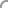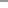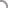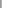Welcome Guest User
 • Spotlight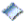New Worksheets...Easter Worksheets...Custom Search
 Pre-made Worksheets: By Grade  — Pre-K and Kindergarten  — 1st Grade  — 2nd Grade  — 3rd Grade  — 4th Grade  — 5th Grade   By ThemeValentine's DaySt. Patrick's DayEasterSpringSummerFallHalloweenThanksgivingChristmasNew Year   By OperationCounting/ Skip CountingComparisonAdditionSubtractionMultiplicationDivisionMatching   By Special Topics/ Skills  — Basic Numbers  — Number Lines  — Fact Family  — Place Value   — Time   — Thermometer   — Money  — Even/Odd Numbers   — Roman Numbers  — Prime Numbers  — Sort/Order Numbers  — Measurement  — Color By Number  — Word problems  — Ordinal Number & Names  — Rounding  — Percentage  — Fractions  — Decimals   — Algebra  — Shapes/Geometry  — Math Drills Custom Worksheets: • Standard Operators    Addition/Subtraction    Multiplication/Division • Number Tables • Math Fact Table Interactive Flash Cards • Multiplication • Addition Other Activities • Quiz/Assessments Contact Us • Suggestions Notify Me >> Enter Email: Testimonial ... Fabulous! My son didn't understand Fact Families at school - and after I found your site, he mastered the concept very quickly... Texas, USA Tell us what do you think about our site.>>
 Explore Schools in USA AboutUs.org Page MathCentral4Kids Site Federal Education Regulations California Law on Education Other sites: A B C D FR F G H IAirlines Scottsdale Deals Privacy Policy Terms of Use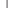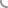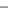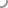Select a Worksheet

Categories:
 1. Prime Numbers 2. Sorting 3. Addition 4. Subtraction 5. Comparison 6. Measurement 7. Fractions 8. Multiplication 9. Division 10. Mixed Operations 11. Shapes/Geometry 12. Rounding 13. Percent 14. Summer Practice Note: - Click here for Spring Math Worksheets.- Click here to create Custom and Mixed Worksheets. - Click here for Math Drills Worksheets.

 1 Prime Numbers Top

Prime Numbers
1 to 100

 2 Sorting Top

Sorting - Whole Numbers, Fractions & Decimals
Ascending
Descending
Mixed - Ascending/Descending

Single Digit - Set 3

Addition - Decimal Numbers
Tenths (Level A)
Hundredths (Level A)

 4 Subtraction Top

Subtract a number
(No Borrowing/Trading) - Single Digit

Subtraction - Decimal Numbers
Tenths (Level A)
Hundredths (Level A)

 5 Comparison Top

Compare Decimal Numbers

Compare Fractions (Simple, Unit Fractions, Proper and Mixed Numbers with like denominators)
Level C1 (Simple)
Level C2 (Medium)
Level C3 (Hard)

 6 Measurement Top

Measurement - Length
U.S. Customary - Line (inches)

 7 Fractions Top

Convert - Fractions to Decimals
Level A1 - Proper Fraction, Denominator 10, 100
Level A2 - Proper/Improper Fractions, Denominator 10, 100, 1000
Level A3 - Proper Fraction, Denominator 2,4,5,10,20,40,50,100 and results in 0.25, 0.5, 0.75

Reduce Fractions to Lowest Terms
Level C1 (Simple)
Level C2 (Medium)
Level C3 (Hard)

Fractions (Like denominators) Addition
Level C1 (Simple)
Level C2 (Medium)
Level C3 (Hard)

 8 Multiplication Top

Multiplication : Single Digit
Tables of 0 to 5 ([0 - 5] X [0 - 9])
Tables of 0 to 6 ([0 - 6] X [0 - 9])
Tables of 0 to 7 ([0 - 7] X [0 - 9])
Tables of 0 to 8 ([0 - 8] X [0 - 9])
Tables of 0 to 9 ([0 - 9] X [0 - 9])

Multiplication : Multi-digit
Level C1 (Simple - 3 digit # x 1 digit #)
Level C2 (Medium - 2 digit # x 2 digit #)
Level C3 (Hard - 3 digit # x 2 digit #)

Multiplication - Basic Drills
One Digit (0 to 9)
One Digit (0 to 12)
1-2 Digits (#'s from 6 to 12)
Tough Ones (Selective #'s from 4 to 12)

Multiplication Estimation
Decimal Numbers

 9 Division Top

Single Digit Divisor, with Remainder
Level 1 (Simple)

Division - Mixed
Whole and Decimal Numbers - Set 1

Division - Basic Drills
Single Digit Divisor, without Remainder

 10 Mixed Operations Top

Drills - Mixed Operators - Addition, Subtraction
Single Digit

Drills - Mixed Operators - Addition, Multiplication
Single Digit

Drills - Mixed Operators - Addition, Subtraction, Multiplication
Single Digit

Drills - Mixed Operators - Multiplication, Division
Single Digit

Drills - Mixed Operators - Addition, Subtraction, Multiplication, Division
Single Digit

 11 Shapes/Geometry Top

Classify shapes
Triangle types

 12 Rounding Top

Round to nearest thousand

Round to nearest tenth

 13 Percent Top

Convert - Percent to Reduced Fractions

Convert Decimals to Percentage

 14 Summer Practice Top

Summer Practice Part 1 - Addition (0 to 9)

Summer Practice Part 2 - Subtraction (0 to 9)

Summer Practice Part 3 - Multiplication (0 to 9)

Summer Practice Part 4 - Division (0 to 9)

Summer Practice Part 5 - Addition (0 to 12)

Summer Practice Part 6 - Subtraction (0 to 12)

Summer Practice Part 7 - Multiplication (0 to 12)

Summer Practice Part 8 - Division (0 to 12)

 Want to get notified about new myWeblets worksheets?
Search for Worksheets: